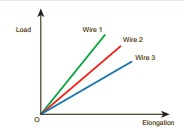# TN 11TH PHYSICS - Online Test

Q1. A ball of mass 1 kg and another of mass 2 kg are dropped from a tall building whose height is 80 m. After, a fall of 40 m each towards Earth, their respective kinetic energies will be in the ratio of
Explaination / Solution:
No Explaination.

Q2. A couple produces,
Explaination / Solution:
No Explaination.

Q3. A planet moving along an elliptical orbit is closest to the Sun at distance r1 and farthest away at a distance of r2. If v1 and v2 are linear speeds at these points respectively. Then the ratio V1/V2 is
Explaination / Solution:
No Explaination.

Q4. The load – elongation graph of three wires of the same material are shown in figure. Which of the following wire is the thickest?Explaination / Solution:
No Explaination.

Q5. When a cycle tyre suddenly bursts, the air inside the tyre expands. This process is
Explaination / Solution:
No Explaination.

Q6. An ideal gas is maintained at constant pressure. If the temperature of an ideal gas increases from 100K to 1000K then the rms speed of the gas molecules
Explaination / Solution:
No Explaination.

Q7. The length of a second’s pendulum on the surface of the Earth is 0.9 m. The length of the same pendulum on surface of planet X such that the acceleration of the planet X is n times greater than the Earth is
Explaination / Solution:
No Explaination.

Q8. For a particular tube, among six harmonic frequencies below 1000 Hz, only four harmonic frequencies are given : 300 Hz, 600 Hz, 750 Hz and 900 Hz. What are the two other frequencies missing from this list?.
Explaination / Solution:
No Explaination.

Q9. If the length and time period of an oscillating pendulum have errors of 1% and 3% respectively then the error in measurement of acceleration due to gravity is
Explaination / Solution:
No Explaination.

Q10. Which one of the following physical quantities cannot be represented by a scalar?
Explaination / Solution:
No Explaination.